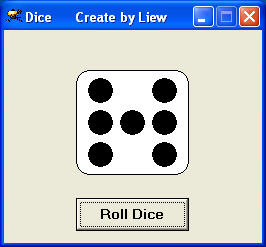# A Dice

This program creates a die which can be used to play board games. It can also be incorporated into VB games that require a dice. VB games that you can create in Visual Basic are step and ladder game, monopoly and more. First , you draw a rounded square in the project windows. Secondly, you need to draw an array of 7 dots and VB will automatically labeled them as shape1(0), shape1(1),shape1(2),  shape1(3), shape1(4), shape1(5) and shape1(6). You can control the appearance of the dots using the random function Rnd.### The Code

```Private Sub Command1_Click()

n = Int(1 + Rnd * 6)
For i = 0 To 6
Shape1(i).Visible = False
Next

If n = 1 Then
Shape1(3).Visible = True
End If

If n = 2 Then
Shape1(2).Visible = True
Shape1(4).Visible = True
End If

If n = 3 Then
Shape1(2).Visible = True
Shape1(3).Visible = True
Shape1(4).Visible = True
End If

If n = 4 Then
Shape1(0).Visible = True
Shape1(2).Visible = True
Shape1(4).Visible = True
Shape1(6).Visible = True
End If

If n = 5 Then
Shape1(0).Visible = True
Shape1(2).Visible = True
Shape1(3).Visible = True
Shape1(4).Visible = True
Shape1(6).Visible = True
End If

If n = 6 Then
Shape1(0).Visible = True
Shape1(1).Visible = True
Shape1(2).Visible = True
Shape1(4).Visible = True
Shape1(5).Visible = True
Shape1(6).Visible = True
End If

End Sub